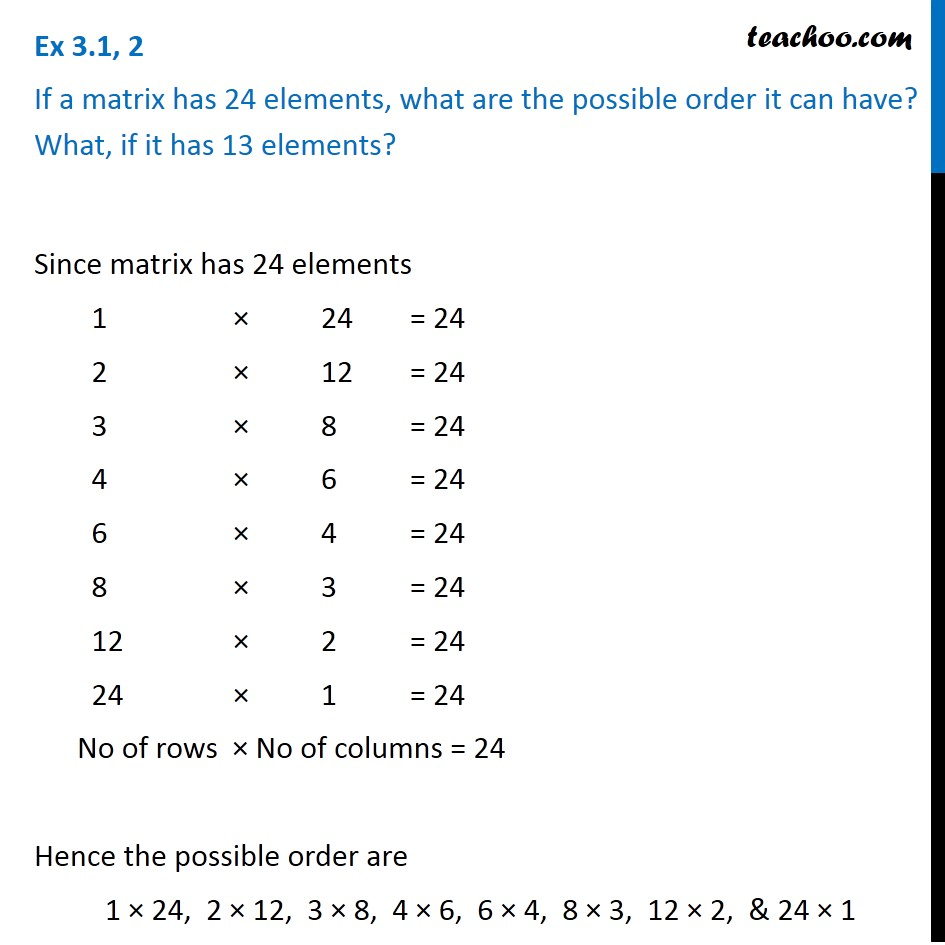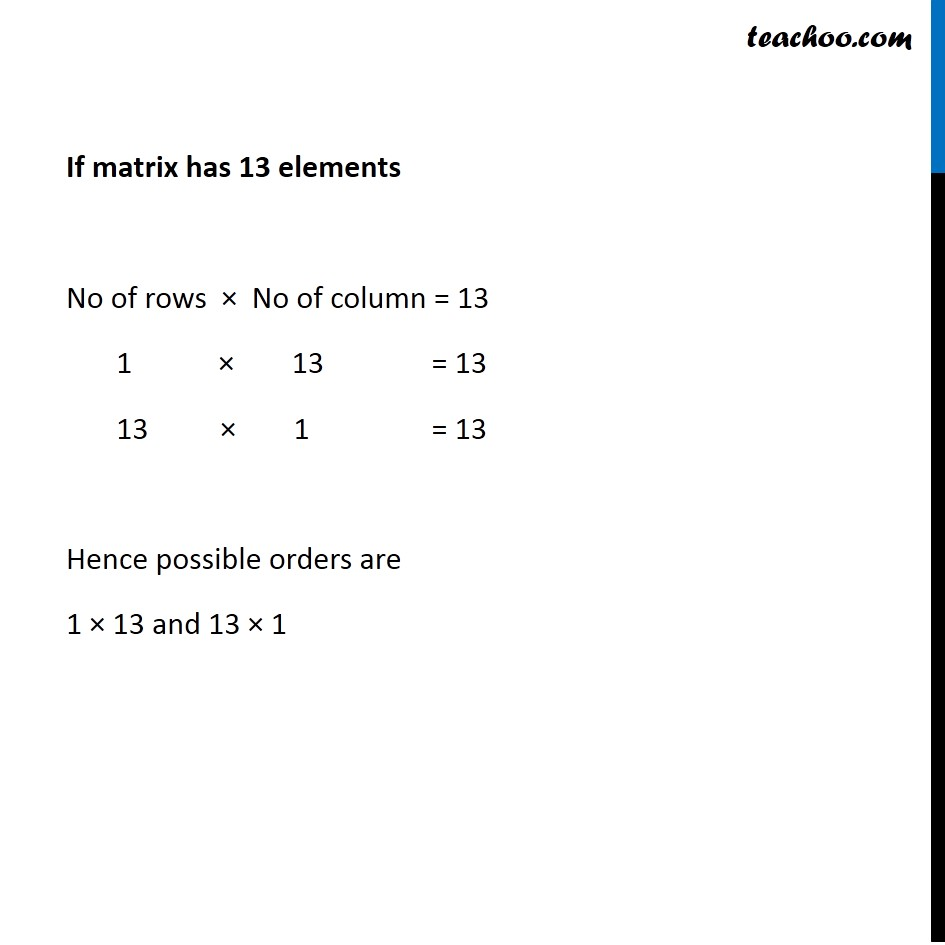1. Chapter 3 Class 12 Matrices (Term 1)
2. Concept wise
3. Formation and order of matrix

Transcript

Ex 3.1, 2 If a matrix has 24 elements, what are the possible order it can have? What, if it has 13 elements? Since matrix has 24 elements 1 × 24 = 24 2 × 12 = 24 3 × 8 = 24 4 × 6 = 24 6 × 4 = 24 8 × 3 = 24 12 × 2 = 24 24 × 1 = 24 No of rows × No of columns = 24 Hence the possible order are 1 × 24, 2 × 12, 3 × 8, 4 × 6, 6 × 4, 8 × 3, 12 × 2, & 24 × 1 If matrix has 13 elements No of rows × No of column = 13 1 × 13 = 13 13 × 1 = 13 Hence possible orders are 1 × 13 and 13 × 1